﻿ Expressions

User Guide > Working with objects and properties > Types of objects > Variables and Expressions > Expressions

The value of a variable can hold numbers, strings or Boolean values. But it also can hold an expression. Expressions are combinations of constants, variables, mathematical, string or Boolean operators, parentheses and functions. Each expression evaluates to some result.

Here are some examples of expressions:

2 + 3 (Two constants, 2 and 3, are separated by the plus operator)

250 +  Var1 (One constant, 250, adds to the Var1 variable)

“Hello” + “,” + “World!” (Three string constants are concatenated together)

Var1 * Avg(Var2 / 3) (Two variables and a constant in a math expression involving the Avg function)

The program automatically parses the expression and finds variables and functions using the following rules. Any combination of symbols enclosed to quotes is considered a string constant. A valid combination of numbers, the minus symbol and the decimal separator symbol is considered a numeric constant. A combination of symbols and numbers without quotes is considered a variable name. Valid variables are highlighted with blue in the Expression Editor; red means such variable is not found.

The value of an expression can be assigned to any variable. However, the result of expression evaluation differs depending on the type of that resulting variable. For example, the expression ”234” + 56 evaluates to 23456 if the resulting variable is String, but it evaluates to 290 if the resulting variable is Numeric.

To insert a variable into the expression box you can either type its name, or use the variable selection button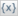.

The list of supported operators

1. Arithmetic Operators

Arithmetic operators are used to perform mathematical operations on numbers. The following mathematical operators are supported:

- (subtraction)

* (multiplication)

/ (division)

% (value percent)

% %(modulo)

^ (exponentiation)

unary - (negation)

Here are some examples:

5+2 = 7

100%3 = 3

5%%2 = 1

Note: You can use + operator to concatenate two and more string value.

"Hello"+","+"Joe" = "Hello,Joe"

2. Relational Operators

Relational operators allow you to compare how one value relates to another. The following relational operators are supported:

> (greater-than)

< (less-than)

<= (less-than or equal to)

>= (greater than or equal to)

!= or <> (not equal to)

= or == (equal)

All of the relational operators can be applied to any two numbers. All other values can only use == or <> operators to see if they are equal or not equal.

Relational operators return Boolean values (true or false). For example:

10 > 20   =  false

"abc"=="abc" = true

"abc"<>"abc" = false

One important point to mention is that the == and <> operators test for complete equality, which means that any string comparisons done with those operators are case sensitive. For example:

"abc"=="abc" = true

"abc"=="ABC" = false

3. Logical Operators

Logical operators are used to perform Boolean operations on Boolean values. The following logical operators are supported:

&& (only true if both values are true)

|| (true if either value is true)

! (returns the opposite of the value)

For example:

true && false = false

true || false = true

!true = false

4. Functions with one argument f(x)

 sqr square sqrt square root abs absolute value sign sign (-1,0,+1) int integer part round nearest integer ceil smallest integer >= x mod compute integer remainder rand get pseudorandom number pi return PI=3.1415926

5. Functions with several arguments f(x1,x2,...)

 avg arithmetic mean = sum/N min minimal value max maximal value

For example:

min(123, 234) = 123

abs(-222)  = 222

Operator Precedence

Operators are said to have precedence, which is a way of describing the rules that determine which operations in a series of expressions get performed first. A simple example would be the expression 2 + 3 * 5. The multiply (*) operator has higher precedence than the add (+) operator, so this expression is equivalent to 2 + (3 * 5). In other words, the expression 3 * 5 is performed first, and then 2 + 15 is performed, resulting in the final value 17.

You can override the natural order of precedence by using parentheses. For instance, the expression (2 + 3) * 5 resolves to 25. Essentially, the sub-expression 2 + 3 is evaluated first, and the result is then used in the expression 5 * 5.

Operator precedence follows the following order, from lowest to highest priority:

&& ,  ||
< , > , <= , >= , != , < > , == , =
+ , -
* , /  , % , %%
!, -(unary)
^

( ), functions

Expression Editor

Autoplay Menu Designer features the built-in expression editor that highlights variable names and calculates expression values. The expression editor looks as follows: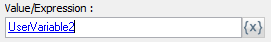You can type expressions directly into the edit box. To insert a variable name into the box, click thebutton. Then select a variable from the object tree window.

In an expression, numbers and string constants are black, functions are maroon, recognized variables are blue and non-recognized variables or combinations of symbols are red.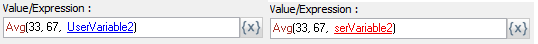You can also see the expression value in the calculated result box, and values of certain variables when you point them in the editor with your mouse.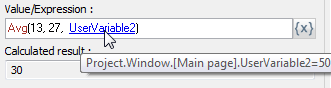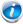Note: run-time values of expressions and variables may differ from those in the design-time.